Algebra 1 section of MathBitsNotebook.comPractice Test 1 - CCSS Algebra 1 Interactive Version - Long ResponseDirections:  Questions for Parts II, III, and IV are long response questions. You will need paper, pencil and a graphing calculator to work out the problems. Check your answer after solving each problem by clicking the ANSWER button.
Part II: (2 pts)
 25. Write the equation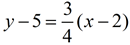in the equivalent form most appropriate for identifying the slope and the y-intercept of the function.
 26. The graph of y = x2 is shown (the solid drawing) at the right. Write the equation for the graph of the transformation of this function (the dotted drawing) which shifts the function three units to the left and one unit up.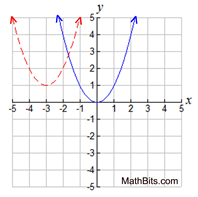27. Explain why a function has only one y-intercept.
 28. Factor completely: 10x2- 8x - 2
 29. Solve for x: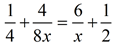30. Express the area of a rectangle with length (4x3 + 2x2 + x + 1) and width (x2 - 3). (Express answer in expanded form.)
 31. A small theater has 15 seats in the first row, 24 seats in the second row, and 33 seats in the third row. The number of seats in each row continues increasing in this same pattern for a total of 6 rows in the theater. How many seats are in the theater?
 32. Find the 8th term of the sequence defined as: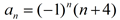Part III:
(4 pts)
 33. Taylor places a one inch frame around the picture taken of her competition win. The height of the picture, with the frame, is represented by x + 4, and the width is represented by x + 2. a)  Express, in terms of x, the height and width of the picture, without the frame, in inches. Label each answer. b)  Express, in terms of x, the area of the picture, without the frame, in square inches. c)  Express, in terms of x, the area of the picture and the frame together, in square inches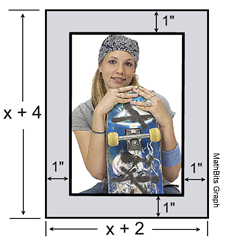34. Kesha has a prepaid phone plan that charges 18¢ for each text message and 15¢ per minute for calls. a)  Write an equation for the cost, C, of sending  t  text messages and talking for  m  minutes. b)  Kesha has \$10 left on her plan, and wants to send 30 text messages.  How much money will remain, if any, on her plan after she sends the 30 texts?
 35. The length of the shortest side of a right triangle is 6 inches.  The lengths of the other two sides are consecutive even integers. a)  Write an equation that can be used to find the lengths of the other two sides of the triangle. b)  Solve the equation for the two missing sides
 36. Average weekly temperatures in central New York were recorded beginning April 1st, as shown. a)  Find a linear regression equation for this data. Round all coefficients to nearest hundredths. b)  Based upon your regression equation, predict the average weekly temperature 10 weeks after April 1st (to nearest degree).    c)  The graph of the residuals for this data is shown below.  What are the residuals telling you about your linear regression equation?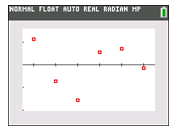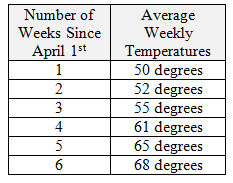Part IV:
(6 pts)
 37. While jogging, Kevin turns his head to see a friend waving from a passing car. Unfortunately, he did not notice that he was jogging directly into a five foot tall roadside delimiter marker.  After hitting the post, Kevin falls to the ground where his front tooth is dislodged and flies upward into the air.  The height attained by the tooth during its airborne journey is shown in the graph at the right.  The x-axis represents the time in seconds and the y-axis the height in feet.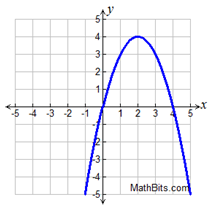a)  The tooth’s journey is a transformation of the function y = x2.  Write an equation to represent the tooth’s journey. b)  Based upon the information given, place constraints upon your new equation so the graph depicts only the actual heights attained by the tooth. c)  What was the maximum height reached by the tooth? d)  During what interval was the height of the tooth decreasing? e)  How long did it take for the tooth to return to the ground?